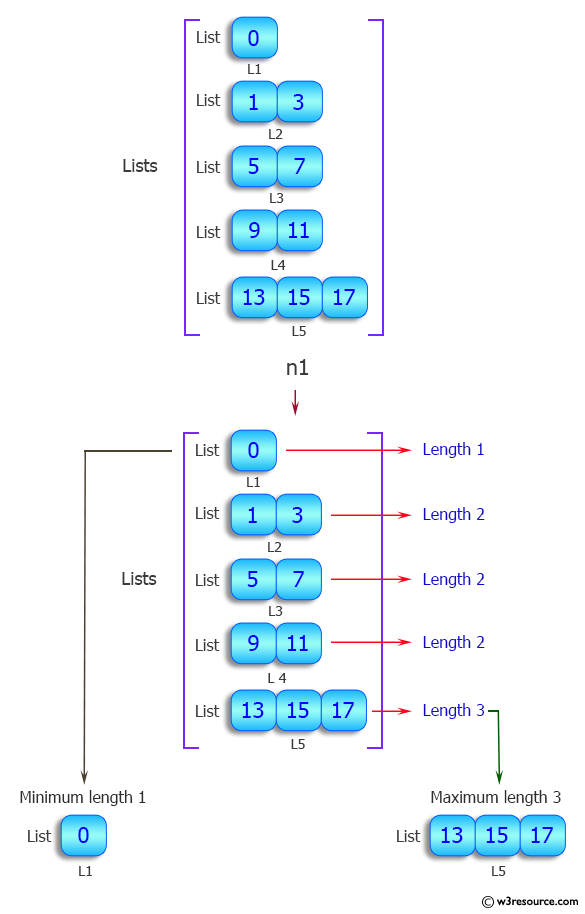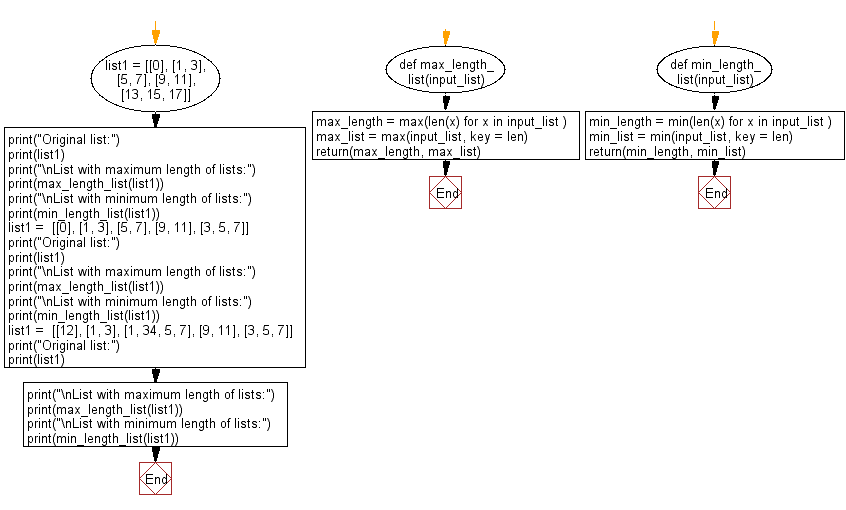﻿ Python: Find the list with maximum and minimum length - w3resource# Python: Find the list with maximum and minimum length

## Python List: Exercise - 91 with Solution

Write a Python program to find the list with maximum and minimum length.

Sample Solution:

Python Code:

``````def max_length_list(input_list):
max_length = max(len(x) for x in input_list )
max_list = max(input_list, key = len)
return(max_length, max_list)
def min_length_list(input_list):
min_length = min(len(x) for x in input_list )
min_list = min(input_list, key = len)
return(min_length, min_list)
list1 = [, [1, 3], [5, 7], [9, 11], [13, 15, 17]]
print("Original list:")
print(list1)
print("\nList with maximum length of lists:")
print(max_length_list(list1))
print("\nList with minimum length of lists:")
print(min_length_list(list1))
list1 =  [, [1, 3], [5, 7], [9, 11], [3, 5, 7]]
print("Original list:")
print(list1)
print("\nList with maximum length of lists:")
print(max_length_list(list1))
print("\nList with minimum length of lists:")
print(min_length_list(list1))
list1 =  [, [1, 3], [1, 34, 5, 7], [9, 11], [3, 5, 7]]
print("Original list:")
print(list1)
print("\nList with maximum length of lists:")
print(max_length_list(list1))
print("\nList with minimum length of lists:")
print(min_length_list(list1))
```
```

Sample Output:

```Original list:
[, [1, 3], [5, 7], [9, 11], [13, 15, 17]]

List with maximum length of lists:
(3, [13, 15, 17])

List with minimum length of lists:
(1, )
Original list:
[, [1, 3], [5, 7], [9, 11], [3, 5, 7]]

List with maximum length of lists:
(3, [3, 5, 7])

List with minimum length of lists:
(1, )
Original list:
[, [1, 3], [1, 34, 5, 7], [9, 11], [3, 5, 7]]

List with maximum length of lists:
(4, [1, 34, 5, 7])

List with minimum length of lists:
(1, )
```

Pictorial Presentation:Flowchart:## Visualize Python code execution:

The following tool visualize what the computer is doing step-by-step as it executes the said program:

Python Code Editor:

Have another way to solve this solution? Contribute your code (and comments) through Disqus.

What is the difficulty level of this exercise?

Test your Python skills with w3resource's quiz

﻿

## Python: Tips of the Day

Floor Division:

When we speak of division we normally mean (/) float division operator, this will give a precise result in float format with decimals.

For a rounded integer result there is (//) floor division operator in Python. Floor division will only give integer results that are round numbers.

```print(1000 // 300)
print(1000 / 300)```

Output:

```3
3.3333333333333335```